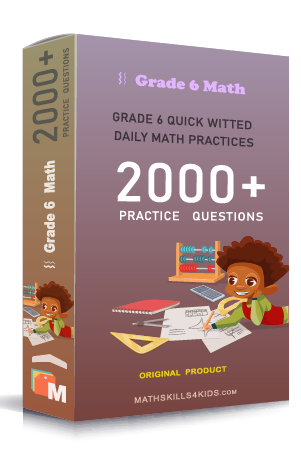# Grade 6 Ratio and rates worksheets with solutions

Subject
Math

Resource Type
Worksheets, Printables, Homeschool

Standards
CCSS 6.RP.A.1 | CCSS 6.RP.A.2 | CCSS 6.RP.A.3

Format
PDF (25.6 MB | 36 pages → Exercises + Solutions)

• ### -15% OFF Over \$100

Promo Code: TOPLA

After Purchase

\$3.85

This package allows you to practice the following skills

Write a ratio

Write a ratio using a fraction

Write a ratio using fraction word problems

Identify equivalent ratio

Write equivalent ratio

Complete a ratio table

Solve equivalent ratios word problems

Find unit rates

Find equivalent rates

Compare ratios word problems

Solve unit rates word problems

Do the ratios from a proportion

Solve the proportion

This product is a part of the Mega Pack
* Grade 6 quick-witted daily math practices *Identify proportional relationships from graphs

Identify proportional relationships from tables

Identify proportional relationships by graphing

Interpret graphs of proportional relationships

Solve scale drawing word problems